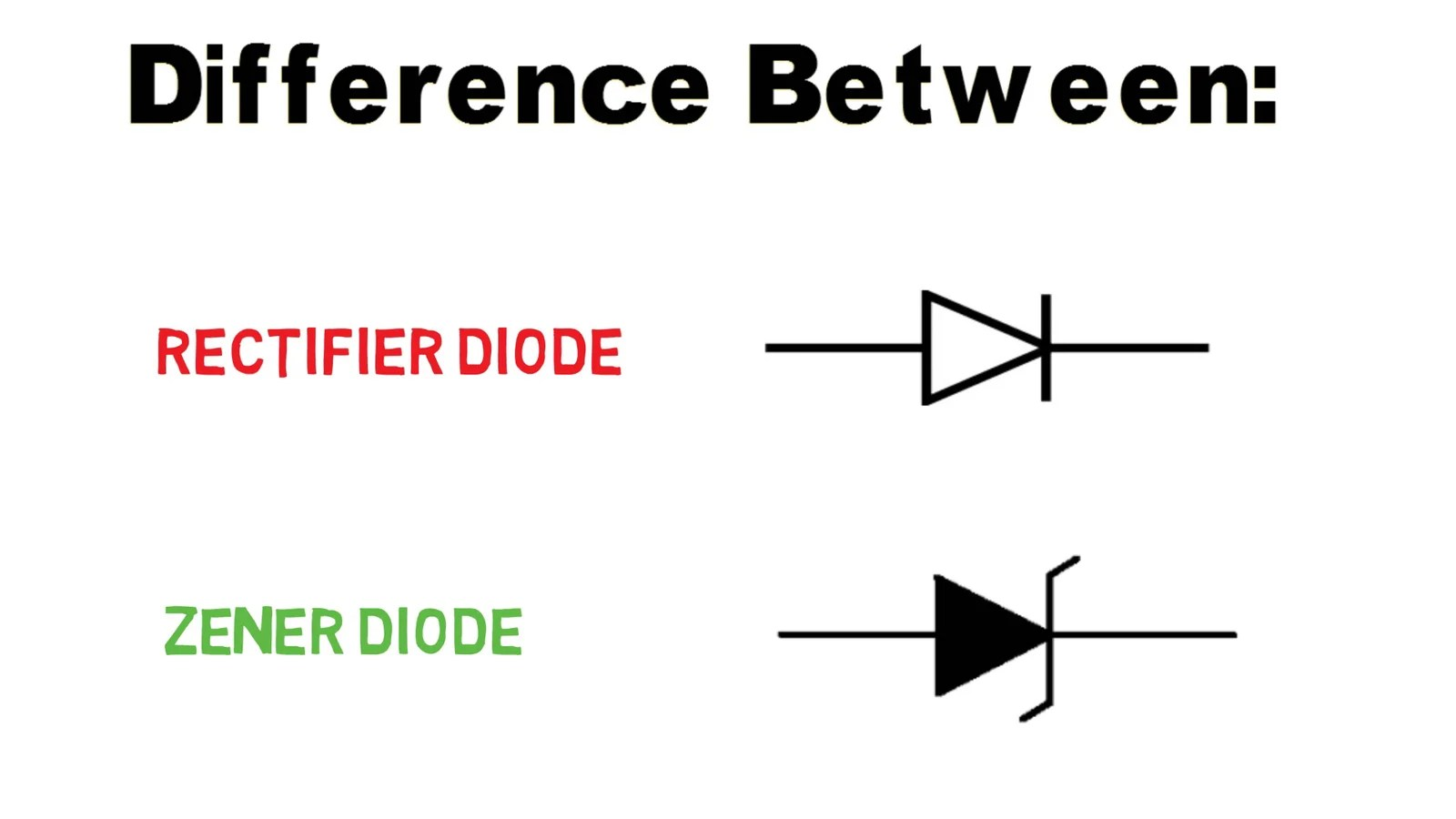# Difference between Zener diode and normal Rectifier diode

A diode is a passive device which allows the flow of current in one direction only. But since there are so many types of a diode, how to differentiate between them and most importantly which one to use according to its requirement in our circuit. “Difference between Zener diode and normal Rectifier diode

So in this post, we are going to see the basic difference between two of the most widely used diodes: Normal rectifier diode and a Zener diode.

Before jumping to its type, let’s see some basic specifications of a diode:
1. (Vf): indicates fwd voltage drop when current flows from p to n terminal
2. (If): is the maximum fwd current a diode can handle
3. (Vr): is the reverse break down voltage when current flows from n to p terminal
4. (Ir): The amount of current that flows when a diode is reverse biased

5. (Reverse recovery time):

When a diode is switched off suddenly, the fwd current flowing through the diode takes a small amount of time to die down and this time is called Reverse Recovery Time.

1. Rectifier Diode:

• A rectifier diode is the simplest p-n junction diode, used mostly for rectification purposes in a half bridge and full bridge rectifier. And that’s because of its high break down voltage, typically of the order of 200 to 1000 volts which is obvious.
• The forward voltage drop (Vf) of a rectifier diode is between .7 to .9 volt.

• As an example, let’s say you want to design a bridge rectifier for your ac to dc converter project. For this bridge rectifier, diode of 1N4 rectifier series is an optimum choice.

2. Zener Diode:

• A Zener diode is made up of p-n junction but heavily doped compared to a normal diode. As a result, it can undergo break down without being damaged.
• And Due to this property only, Zener is used as a voltage regulator in electronic circuits.
• In fact, Zener diodes are never used for rectification purposes.

• Here’s a circuit in which Zener diode is used to prevent the MOSFET gate from destruction by clipping off voltage. The breakdown voltage of this Zener is 5.1 v. Now if accidentally voltage at the gate of MOSFET exceeds 5v, diode breakdown and all current flows through the diode to ground. Thus preventing the MOSFET from any kind of destruction.

• Here’s another circuit in which two diodes are connected facing each other’s p terminal. If an A.C signal is given at input, one diode clips of voltage in positive half whereas other in negative half and thus we get voltage under the specified limit in both half cycle of a.c.

• As an example, I used 5V Zener in the project “ Digital voltmeter using Arduino” in which it is connected across the capacitor to prevent Arduino in case voltage at its analog pin exceeds 5v.
1.Hassan Guttirez says: